## Saturday, 31 March 2012

### Basics of Integration. Part I. Technical basis.

Unlike the passages I've written before, I hope this one serves as a basic theory on integration. We may discuss more technical issue in a different approach than the textbook.

I assume some basic knowledge on differentiation and basic algebra, while some good basic knowledge on real analysis like technical refining on Riemann sum, MVT (mean value theorem) or upper/lower limit of Riemann sum, are not assumed but maybe discussed a bit here. The issue discussed will be less vigour then undergrad. course.

We start with FToC (fundamental theorem of calculus), anti-derivatives, then some technical work on substituion and by part; some real application on area and volume. That should be enough to cover most A-level techniques. The application goes deeper for differential equations, some simple contour integration, integration on polar coordinates and multiple integral. To end with we will focus on the art of integration, some special and beautiful techniques which kills many ugly integrands. Questions will be from A-level materials and the series "Today's calculation of Integral" (TCoI).

Infinitesimal

Strictly speaking, infinitesimal is a series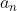so that for every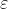larger than zero exist N so that for all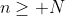we have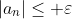, then by definition of limit, the sequence tends to zero.

However, this definition is quite academic and we may want to make it easier. A easy interpretation is that, infinitesimal is a quantity that "infinitely close to zero but non-zero". If infinitely large on a real number line is well-defined, we can say infinitesimal is the reciprocal of infinitely large numbers.

In differentiation, we have learnt the first principle on differentiation, showing that x-change in "infintiely small interval" dividing y-change in "corresponding interval" equals to the instantaneous slope. The term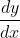developed by Leibniz comes from the infnitesimal, and in fact, dy and dx are infinitesimals and is an existing quantity. That's why we can "multiply dx on both sides" and "squaring dx" because a real quantity is commutative in common calculation. Of course, we need a vigour proof for that issues and that's beyond our scoop.

The relationship between infinitesimal and integration is as follows.

Notation. In the following sections,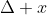represents an infinitesimal interval in x. In calculation, the quantity of a single infinitesimal quantity is useless, but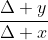can be stated aswhich is the slope of the curve.

Riemann sum as the definition of integration

In this level we are not going to discuss different definition of integration (unlike differentiation, integration has many different valid definition) like Lebesgue integration because that's far too difficult. Instead we turn it into a reality concept.

Imformal definition.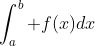is defined as the area between f(x)and the x-axis from a to b (i.e., enclosed by two line x=a and x=b as well).

Definition. (Riemann integral) For a function f, define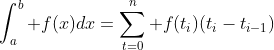We divide a to b into n (not necessarily equal) parts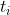, the term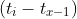is the x-change whileis the corresponding y-change. Multiplying them gives a rectangle under the curve. If the division of part is finite, we get an estimation of integral (in which we will discuss later), and if the parts becomes infinitesimals (divided into infinitely many parts), the value tends to the real area and hence the value of integral is given. In the infinitesimal case, we have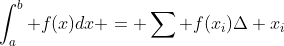Fundamental theorem of calculus (FToC)

FToC I: Let f be function in close interval [a,b], define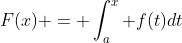, then F(x) can be differentiated in [a,b], and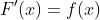for all x in [a,b].

FToC II: Let F'(x) = f(x). Then.

The proof of FoTC I used MVT (mean value theorem) in the vigorous proof, and here is an easier proof (which should be avoided in higher level):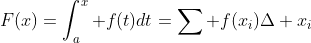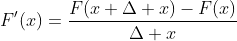Whereis an infinitesimal interval, which has the same effect as limiting to zero.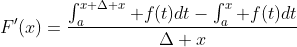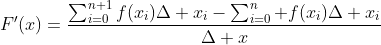We can assume that the first integrands has only one extra term because it only has a difference of. We now we have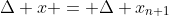.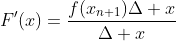If an appropiateis to be added so that the integrand is correct, it lies somewhere between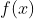and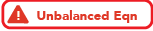referred to Mean Value Theorem. Whensqueezes to zero,squeezes to.
Therefore we haveWe shall prove FToC II by the concept of infinitesimal (instead of limit language in real analysis) because the idea is same as definite integral later.

Let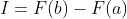. Now notice that I is the corresponding y-change of F from a to b. Rewrite I as: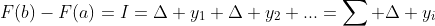, where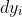is the corresponding y-change of F in an infinitesimal interval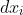. The overall sum describes the change in the interval [a.b].

Here is an analogy to make the above statement easier to understand. Let f(x) = 2x. It's clear that f(3) - f(1) = 4. The "y-change of f from 1 to 3 is +4". Now we make things smaller:

"y-change of f from 1 to 1.5 is +1", similarly, "y-change of f from 1.5 to 2 is +1" and so on. If we add them up we can still get the same result.
Now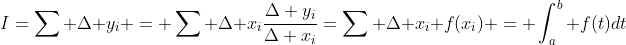.

Warning. I shall reaffrim here that the above proof is informal, but the idea is the same.

Indefinite integral and uniqueness

Definition. Indefinite integral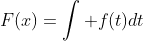of f, is the anti-derivative of f. i.e.,for all defined domain of F. In forms of definite integral,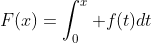.

We now show that integrand of each function is unique except a different constant at the back.

Assume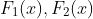are two integration result from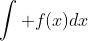.
Since integration is the inverse of differentiation, we have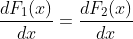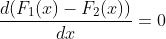, it is clear that only constant's detivative is equal to zero, so we are done.

Significance:
1) As stated, integration and differentation is the inverse each other. i.e.,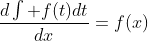and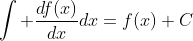(for all integrable and differentiable points on f, respectively), note that after integration there's a constant. Note that this may not work for multivariate function, we are dealing with 1 variable now only.
2) That is, if we can find F so that F' = f, then integrate f gives F. We can solve many simple integrands by such "experiences".

We have now clerify the technical problems that we need in our current level so that we can head into some practical work next time. For those who is not capable to realize the technical problems, especially FoTC, should try to memorize the fact that integration is the inverse of differentiation, and come back after some basic real analysis knowledge.